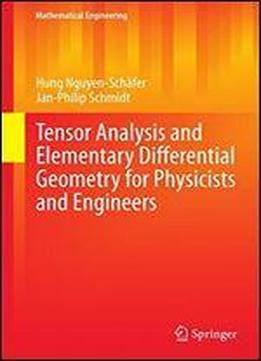Tensor Analysis And Elementary Differential Geometry For Physicists And Engineers (mathematical Engineering) by Hung Nguyen-Schafer / 2014 / English / PDF

Tensors and methods of differential geometry are very useful mathematical tools in many fields of modern physics and computational engineering including relativity physics, electrodynamics, computational fluid dynamics (CFD), continuum mechanics, aero and vibroacoustics, and cybernetics. This book comprehensively presents topics, such as bra-ket notation, tensor analysis, and elementary differential geometry of a moving surface. Moreover, authors intentionally abstain from giving mathematically rigorous definitions and derivations that are however dealt with as precisely as possible. The reader is provided with hands-on calculations and worked-out examples at which he will learn how to handle the bra-ket notation, tensors and differential geometry and to use them in the physical and engineering world. The target audience primarily comprises graduate students in physics and engineering, research scientists, and practicing engineers.

views: 673